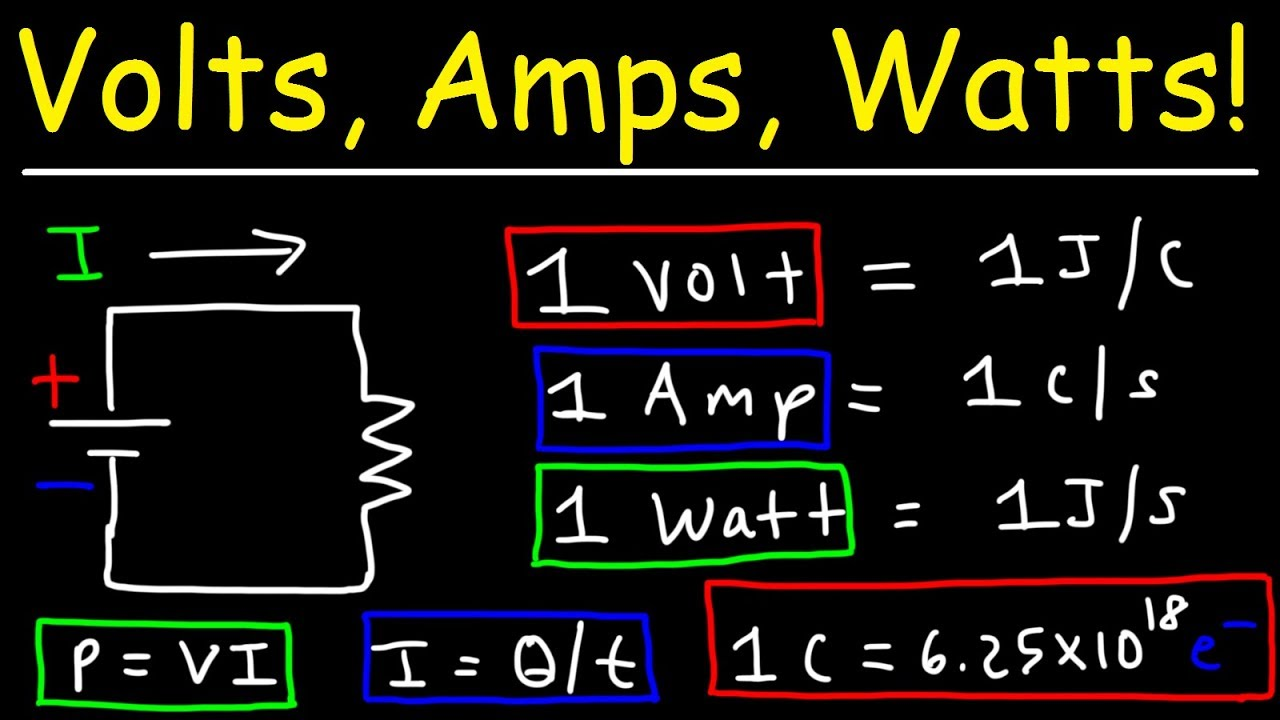## Amps, Volts, Watts, Ohms What are they and how are they different?

Amps, volts, watts, ohms, are four quite important terms within the world of electronics. To begin to understand what they are, it must be known that in electricity 3 basic elements are handled, which are, voltage, current and resistance, which have a lot to do with the terms already mentioned.

## Definition of ampere

Also called ampere, it can be defined as a unit that is responsible for measuring the level of intensity present in the electric current. It received its name in memory of the physicist Ampére.

This unit, as has been said, is responsible for measuring the constant intensity of the current that is maintained in 2 similar conductors and that are located at a distance of one meter.

It is considered as a basic module, in conjunction with units such as the meter for distances, the second for time and kilograms for weight. Furthermore, it can be said that its exact definition does not depend on the amount of electric charge.

## Concept of volts

It is the unit of measurement of voltage. It owes its name to the chemist and physicist of Italian origin Alessandro Volta, who was the creator of the first chemical battery and the voltaic battery.

On the other hand, it could also be said that it is the discrepancy of the potential through a conductor. That is, from when the current of an ampere executes a watt of power. Or between the difference that exists between two points according to the work that is done.## What are watts?

Like amps, volts, and ohms, watts are part of the International Systems unit for power.

It is normally used to weight the rate at which energy is transferred. It can be said then that this unit, its components and subcomponents can be applied to any power. Either at the level of electricity, mechanics, or any other.

The concept of this unit will depend largely on the equations that are applied in the basic units of the International System. As they can be in classical mechanics and electromagnetism.

## Definition of ohms

It can be defined as the modulus from the electrical resistance according to the classification of the International System of Units. It owes its name to the physicist Georg Ohm, who was also the creator of Ohm’s Law.

This unit is the electrical resistance that is originated from 2 points of a conductor, which would be one volt.

In symbology, it is represented by the capital Greek letter omega. It can also be determined as the electrical resistance shown by a pilaster composed of mercury, which is about 107 centimeters high with a temperature that is leveled at zero degrees Celsius.

## Ratio between amps, volts, watts, ohms

To understand the relationship of these four terms or their differences, it can be done with a simple example.

Start by imagining a water pipe, in which the voltage would be equal to the water pressure, the current, which is measured in amps, is equal to the flow rate. This would be the volume of water that passes through the duct.

The resistance, which is measured in ohms, is equivalent to the roughness of the duct, that is, the opposition due to friction by the water in the pipe.

According to its definition, it is that you can know that amps, volts, watts, ohms have a lot of relationship. As they are all part of the International System of Units, it could be said that they work in conjunction with each other.

Knowing the difference between these units is a fundamental part of understanding their individual functioning and, later, their dynamics as a whole.